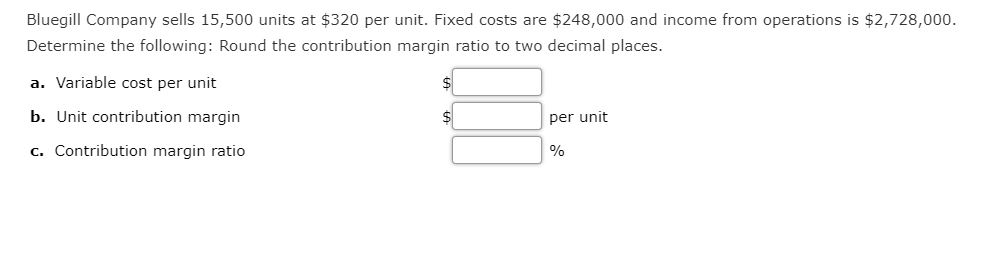# Bluegill Company sells 15,500 units at $320 per unit. Fixed costs are$248,000 and income from operations is $2,728,000. Determine the following: Round the contribution margin ratio to two decimal places. a. Variable cost per unit b. Unit contribution margin per unit c. Contribution margin ratio Questionhelp_outlineImage TranscriptioncloseBluegill Company sells 15,500 units at$320 per unit. Fixed costs are $248,000 and income from operations is$2,728,000. Determine the following: Round the contribution margin ratio to two decimal places. a. Variable cost per unit b. Unit contribution margin per unit c. Contribution margin ratio fullscreen

1 Rating

### Want to see this answer and more?

Experts are waiting 24/7 to provide step-by-step solutions in as fast as 30 minutes!*

*Response times may vary by subject and question complexity. Median response time is 34 minutes for paid subscribers and may be longer for promotional offers.
Tagged in